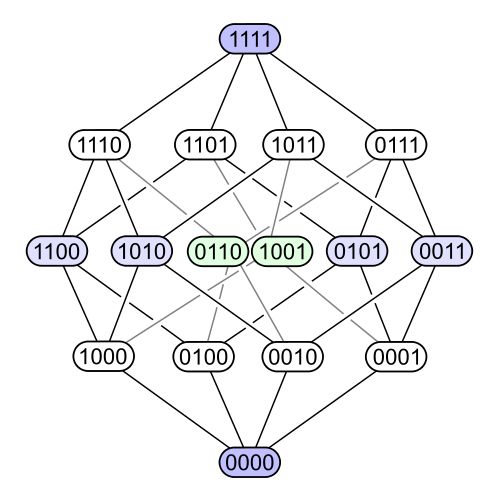Great picture, Fredrick! Yes, a binary relation on \$$S\$$ is the same as a subset of \$$S \times S\$$, so the poset of binary relations on \$$S \$$ is just our friend the power set \$$P(S \times S\$$. So, it looks like an \$$n^2\$$-dimensional cube if \$$S\$$ has \$$n\$$ elements. You're taking \$$n = 2\$$ so your picture looks a lot like this: